# Mechanical Engineering - Engineering Mechanics

### Exercise :: Engineering Mechanics - Section 1

41.

A resultant force is a single force which produces the same effect as produced by all the given forces acting on a body.

 A. True B. False

Explanation:

No answer description available for this question. Let us discuss.

42.

When a rigid body is suspended vertically, and it oscillates with a small amplitude under the action of the force of gravity, the body is known as

 A. simple pendulum B. compound pendulum C. torsional pendulum D. second's pendulum

Explanation:

No answer description available for this question. Let us discuss.

43.

Moment of inertia of a hollow circular section, as shown in the below figure about X-axis, is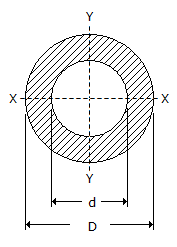A.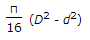B.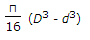C.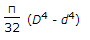D.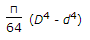Explanation:

No answer description available for this question. Let us discuss.

44.

If two blocks of equal mass are attached to the two ends of a light string and one of the blocks is placed over a smooth horizontal plane while the other is hung freely after passing over a smooth pulley, then the two blocks will have some motion.

 A. Agree B. Disagree

Explanation:

No answer description available for this question. Let us discuss.

45.

During elastic impact, the relative velocity of the two bodies after impact is __________ the relative velocity of the two bodies before impact.

 A. equal to B. equal and opposite to C. less than D. greater than